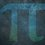# UKMT Special (Problem $22$)

Each of $A$ and $B$ is a $4$-digit palindromic integer.

$C$ is a $3$-digit palindromic integer and

$A - B = C$

What are the possible values of $C$?

[UKMT Hamilton Olympiad $2019$, H$4$]Note by Yajat Shamji
8 months, 2 weeks ago

This discussion board is a place to discuss our Daily Challenges and the math and science related to those challenges. Explanations are more than just a solution — they should explain the steps and thinking strategies that you used to obtain the solution. Comments should further the discussion of math and science.

When posting on Brilliant:

• Use the emojis to react to an explanation, whether you're congratulating a job well done , or just really confused .
• Ask specific questions about the challenge or the steps in somebody's explanation. Well-posed questions can add a lot to the discussion, but posting "I don't understand!" doesn't help anyone.
• Try to contribute something new to the discussion, whether it is an extension, generalization or other idea related to the challenge.

MarkdownAppears as
*italics* or _italics_ italics
**bold** or __bold__ bold
- bulleted- list
• bulleted
• list
1. numbered2. list
1. numbered
2. list
Note: you must add a full line of space before and after lists for them to show up correctly
paragraph 1paragraph 2

paragraph 1

paragraph 2

[example link](https://brilliant.org)example link
> This is a quote
This is a quote
    # I indented these lines
# 4 spaces, and now they show
# up as a code block.

print "hello world"
# I indented these lines
# 4 spaces, and now they show
# up as a code block.

print "hello world"
MathAppears as
Remember to wrap math in $$ ... $$ or $ ... $ to ensure proper formatting.
2 \times 3 $2 \times 3$
2^{34} $2^{34}$
a_{i-1} $a_{i-1}$
\frac{2}{3} $\frac{2}{3}$
\sqrt{2} $\sqrt{2}$
\sum_{i=1}^3 $\sum_{i=1}^3$
\sin \theta $\sin \theta$
\boxed{123} $\boxed{123}$

Sort by:

You have until next Saturday, $3:00$pm!

- 8 months, 2 weeks ago

Rewrite as

   a b b a
c d d c
_______-
e f e


Step 1) Notice that the difference of 2 four digit number is a three digit number, this implies that (look at thousand column) a - c = 0 or a - c - 1 = 0 (in the case of hundred column borrowing from thousands column).

   If a - c = 0, then (look at ones column) e = 0
and implies that the subtraction result is a two digit number which is not true.
Therefore, a - c - 1 = 0   ->   a = c + 1.


Step 2) Notice that (look at ones column) a - c = e or 10 + a - c = e (in the case of hundred column borrowing from tens column).

   Substitute a from step 1.
If 10 + a - c = e, then 10 + a - c = e     ->     10 + c + 1 - c = e     ->     e = 11
However, c is a one digit, so this assumption must be false.
Therefore, a - c = e     ->     c + 1 - c = e     ->     e = 1

a b b a
c d d c
_______-
1 f 1


Step 3) Notice that a = c + 1, however, (look at thousands column) the result is three digit, this implies that b < d and borrow from the thousands column , 10 + b - d = 1 or 10 + b - d - 1 = 1

   If 10 + b - d = 1, this implies that the tens column doesn't borrow, however, b < d,
so this assumption must be false.
Therefore, 10 + b - d - 1 = 1     ->     8 + b = d       ->     b = 0, d = 8  or  b = 1, d = 9
Otherwise, d is a two digit number


Step 4) Notice that, since a = c + 1 the ones column doesn't borrow from the tens column and b < d, therefore 10 + b - d = f (in the case of hundred column borrowing from tens column).

   Substitute b and d from step 3.
Case 1 : b = 0, d = 8
10 + 0 - 8 = f     ->     f = 2
Case 2 : b = 1, d = 9
10 + 1 - 9 = f     ->     f = 2
In either case, f = 2

a b b a
c d d c
_______-
1 2 1


Therefore, C = 121

- 8 months, 2 weeks ago

That's wrong. Try again!

- 8 months, 2 weeks ago

I see, I forgot the carrier. BTW, do you do integral?

- 8 months, 1 week ago

No.

- 8 months, 1 week ago